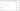← Go BackApril 14, 20202 min read • IN tech

## Neural Net Problems - Exercise 6

Here are my solutions to exercise 6.

## The Backpropagation Algorithm

### Part 1 - Backpropagation with a Single Modified Neuron

#### Question

Suppose we modify a single neuron in a feedforward network so that the output from the neuron is given by $f(\sum_j w_j x_j +b)$, where $f$ is some function other than the sigmoid. How should we modify the backpropagation algorithm in this case?

#### Solution

We first would have to calculate the derviate for the function $f$ since it is needed for the backpropagation output error vector $\delta^L$ and $\delta^l$. But other than that the neural network does not need any tweaking. You may think that it needs tweaking because $\delta_j^l$ is dependent on $f$ but we have defined $\delta_j^l = \frac{\partial C}{\partial z_j^l}$ and $\delta_j^l \ne \frac{\partial C}{\partial a_j^l}$. We did this because it makes our lives easier for this particular case!

From Michael Nielsen:

You might wonder why the demon is changing the weighted input $z_j^l$. Surely it’d be more natural to imagine the demon changing the output activation $a_j^l$, with the result that we’d be using $\frac{\partial C}{\partial a_j^l}$ as our measure of error. In fact, if you do this things work out quite similarly to the discussion below. But it turns out to make the presentation of backpropagation a little more algebraically complicated. So we’ll stick with $\delta_j^l = \frac{\partial C}{\partial z_j^l}$ as our measure of error.

### Part 2 - Backpropagation with Linear Neurons

#### Question

Suppose we replace the usual non-linear $\sigma$ function with $\sigma(z) = z$ throughout the network. Rewrite the backpropagation algorithm for this case.

#### Solution

Since $\sigma(z) = z$ then $\sigma'(z) = 1$, so it follows that $\delta^L = \nabla_a C \circ \sigma'(z^L) = \nabla_a C$. Also, $\delta^l = ((w^{l+1})^T \delta^{l+1}) \circ \sigma'(z^l) = (w^{l+1})^T \delta^{l+1}$.